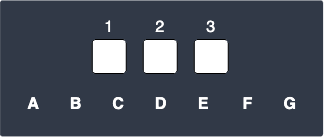search
Search
Map of Data Science
Guest 0reps
exit_to_appLog out
Map of data science
Thanks for the thanks!
close
account_circle
Profile
exit_to_app
Sign out
search
keyboard_voice
close
Searching Tips
Search for a recipe:
"Creating a table in MySQL"
Search for an API documentation: "@append"
Search for code: "!dataframe"
Apply a tag filter: "#python"
Useful Shortcuts
/ to open search panel
Esc to close search panel
to navigate between search results
d to clear all current filters
Enter to expand content previewDoc SearchCode Search BetaSORRY NOTHING FOUND!
mic
Start speaking...Voice search is only supported in Safari and Chrome.
Shrink
Navigate to
A
A
brightness_medium
share
arrow_backShareTwitterFacebook
check_circle
Mark as learned
thumb_up
0
thumb_down
0
chat_bubble_outline
0
auto_stories new
settings

# Comprehensive Guide on Permutations

Probability and Statistics
chevron_right
Probability Theory
schedule Oct 31, 2022
Last updated
local_offer Probability and Statistics
Tags
map
Check out the interactive map of data science

Let's start with a motivating example before we state the formal definition of permutations.

Example.

# Total number of ways to arrange letters

Suppose we have $7$ letters: $\mathrm{A}$, $\mathrm{B}$, $\mathrm{C}$, $\mathrm{D}$, $\mathrm{E}$, $\mathrm{F}$ and $\mathrm{G}$. We select $3$ letters. How many different arrangements are there?

Solution. The word "arrangement" means that the ordering matters. For instance, $\mathrm{ABC}$ is considered to be different from $\mathrm{BAC}$. Let's illustrate the scenario:We have $3$ available slots, and we have to fill each slot with a different letter.

• for the 1st slot, we have 7 letters to choose from.

• for the 2nd slot, since we have already picked a letter for the 1st slot, we only have 6 letters to choose from.

• for the 3rd slot, since two letters have already been chosen, we have 5 letters left to choose from.

The total number of ways to fill the slots is equal to the product of the number of ways to fill each slot:

$$\begin{equation}\label{eq:D3iQ7UTUTFCzzCLKSH6} 7\times6\times5=210 \end{equation}$$

Let's now generalize the calculation by defining the following variables:

• $n$ is the total number of elements to initially choose from.

• $r$ is the number of elements to choose.

In our example, $n=7$ since we have $7$ letters, and $r=3$ because we must choose $3$ letters. Now recall how we calculated the total number of arrangements in \eqref{eq:D3iQ7UTUTFCzzCLKSH6}. Using $n$ and $r$, this number can be computed by:

$$\begin{equation}\label{eq:ioMaMIui2M87EGPWm5o} \frac{n!}{(n-r)!} \end{equation}$$

To understand why this works, let's substitute $n=7$ and $r=3$ as in our example:

$$\frac{7!}{(7-3)!}= \frac{7!}{4!}=7\times6\times5$$

Notice how this is exactly how we computed the total number of arrangements in \eqref{eq:D3iQ7UTUTFCzzCLKSH6}. Mathematicians prefer to use the word "permutations" instead of "arrangements" to emphasize the fact that ordering matters.

Definition.

# Definition and formula for permutation

Permutation refers to the number of ways of ordering $r$ elements from a total of $n$ elements, and is computed by:

$$_nP_r = \frac{n!}{(n-r)!}$$

Where:

• $n$ is the total number of elements.

• $r$ is the number of elements to select.

Let's now go through some examples of applying this formula.

Example.

## Constructing numbers from digits

Suppose we have six digits from $1$ to $6$. How many 3-digit numbers can we construct from these digits if each digit can only be used once?

Solution. Permutation applies in this case because the $3$-digit number $456$ is considered different from $546$, and hence the ordering matters. Let $n=6$ and $r=3$ because we're selecting $3$ digits out of a total of $6$ digits. Applying the formula for permutation gives:

\begin{align*} _6P_3&=\frac{6!}{(6-3)!}\\ &=\frac{6!}{3!}\\ &= 6\times5\times4\\ &= 120 \end{align*}

Therefore, a total of $120$ digits can be constructed.

Example.

## Rearranging balls

If we had $5$ balls numbered from $1$ to $5$, how many different ways could we order exactly two of them?

Solution. Once again, permutation applies because we are concerned with the ordering of the balls. Let $n=5$ and $r=2$ because we have $5$ elements, from which we select $2$ elements. We now apply the formula for permutation:

\begin{align*} _5P_2&=\frac{5!}{(5-2)!}\\ &=\frac{5!}{3!}\\ &=5\times4\\ &=20 \end{align*}

Therefore, there are $20$ ways of ordering $2$ out of $5$ balls.